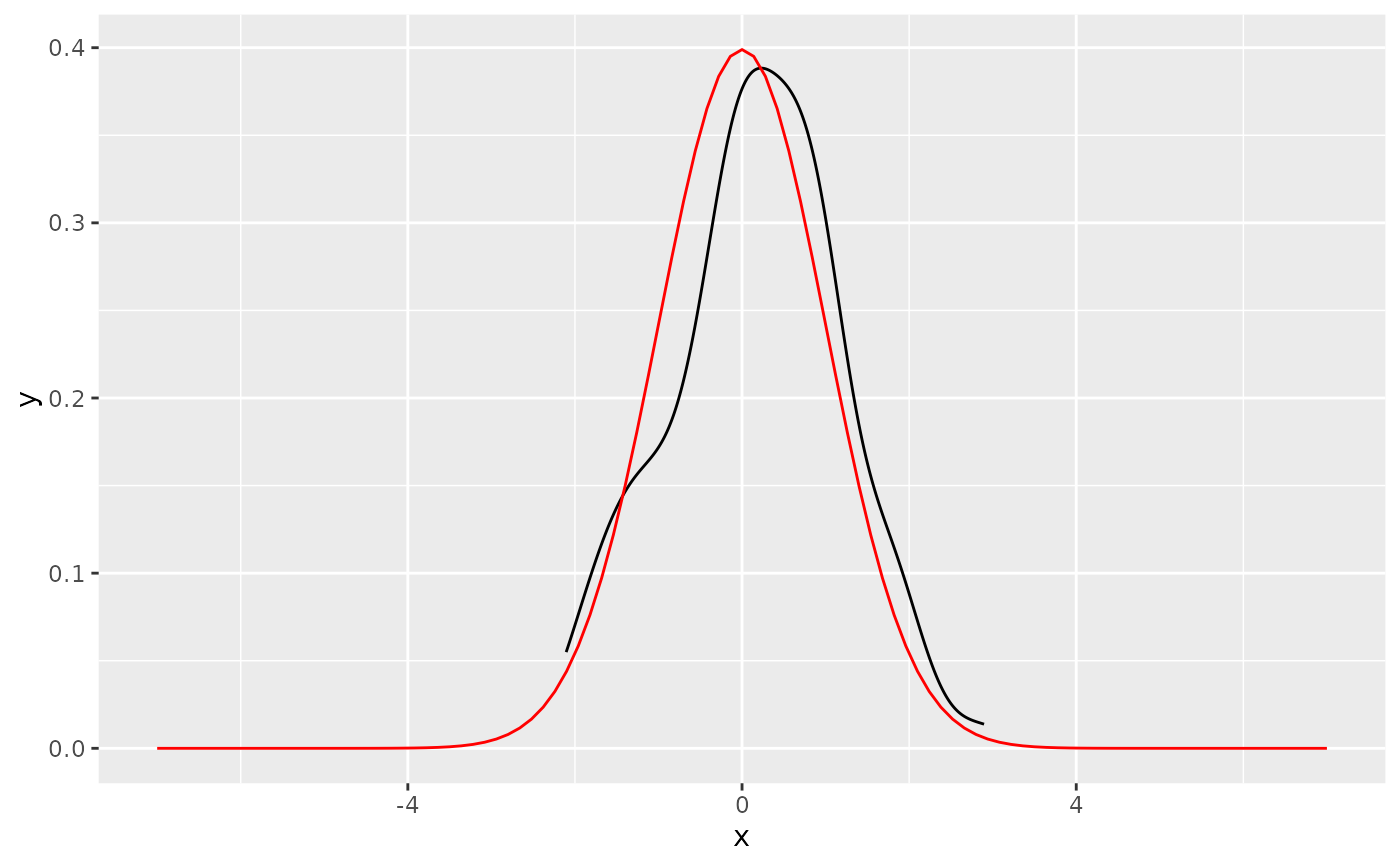Computes and draws a function as a continuous curve. This makes it easy to superimpose a function on top of an existing plot. The function is called with a grid of evenly spaced values along the x axis, and the results are drawn (by default) with a line.

## Usage

geom_function(
mapping = NULL,
data = NULL,
stat = "function",
position = "identity",
...,
na.rm = FALSE,
show.legend = NA,
inherit.aes = TRUE
)

stat_function(
mapping = NULL,
data = NULL,
geom = "function",
position = "identity",
...,
fun,
xlim = NULL,
n = 101,
args = list(),
na.rm = FALSE,
show.legend = NA,
inherit.aes = TRUE
)

## Arguments

mapping

Set of aesthetic mappings created by aes(). If specified and inherit.aes = TRUE (the default), it is combined with the default mapping at the top level of the plot. You must supply mapping if there is no plot mapping.

data

Ignored by stat_function(), do not use.

stat

The statistical transformation to use on the data for this layer, either as a ggproto Geom subclass or as a string naming the stat stripped of the stat_ prefix (e.g. "count" rather than "stat_count")

position

Position adjustment, either as a string naming the adjustment (e.g. "jitter" to use position_jitter), or the result of a call to a position adjustment function. Use the latter if you need to change the settings of the adjustment.

...

Other arguments passed on to layer(). These are often aesthetics, used to set an aesthetic to a fixed value, like colour = "red" or size = 3. They may also be parameters to the paired geom/stat.

na.rm

If FALSE, the default, missing values are removed with a warning. If TRUE, missing values are silently removed.

show.legend

logical. Should this layer be included in the legends? NA, the default, includes if any aesthetics are mapped. FALSE never includes, and TRUE always includes. It can also be a named logical vector to finely select the aesthetics to display.

inherit.aes

If FALSE, overrides the default aesthetics, rather than combining with them. This is most useful for helper functions that define both data and aesthetics and shouldn't inherit behaviour from the default plot specification, e.g. borders().

geom

The geometric object to use to display the data, either as a ggproto Geom subclass or as a string naming the geom stripped of the geom_ prefix (e.g. "point" rather than "geom_point")

fun

Function to use. Either 1) an anonymous function in the base or rlang formula syntax (see rlang::as_function()) or 2) a quoted or character name referencing a function; see examples. Must be vectorised.

xlim

Optionally, specify the range of the function.

n

Number of points to interpolate along the x axis.

args

List of additional arguments passed on to the function defined by fun.

## Aesthetics

geom_function() understands the following aesthetics (required aesthetics are in bold):

• x

• y

• alpha

• colour

• group

• linetype

• linewidth

Learn more about setting these aesthetics in vignette("ggplot2-specs").

## Computed variables

These are calculated by the 'stat' part of layers and can be accessed with delayed evaluation.

• after_stat(x)
x values along a grid.

• after_stat(y)
values of the function evaluated at corresponding x.

rlang::as_function()

## Examples


# geom_function() is useful for overlaying functions
set.seed(1492)
ggplot(data.frame(x = rnorm(100)), aes(x)) +
geom_density() +
geom_function(fun = dnorm, colour = "red")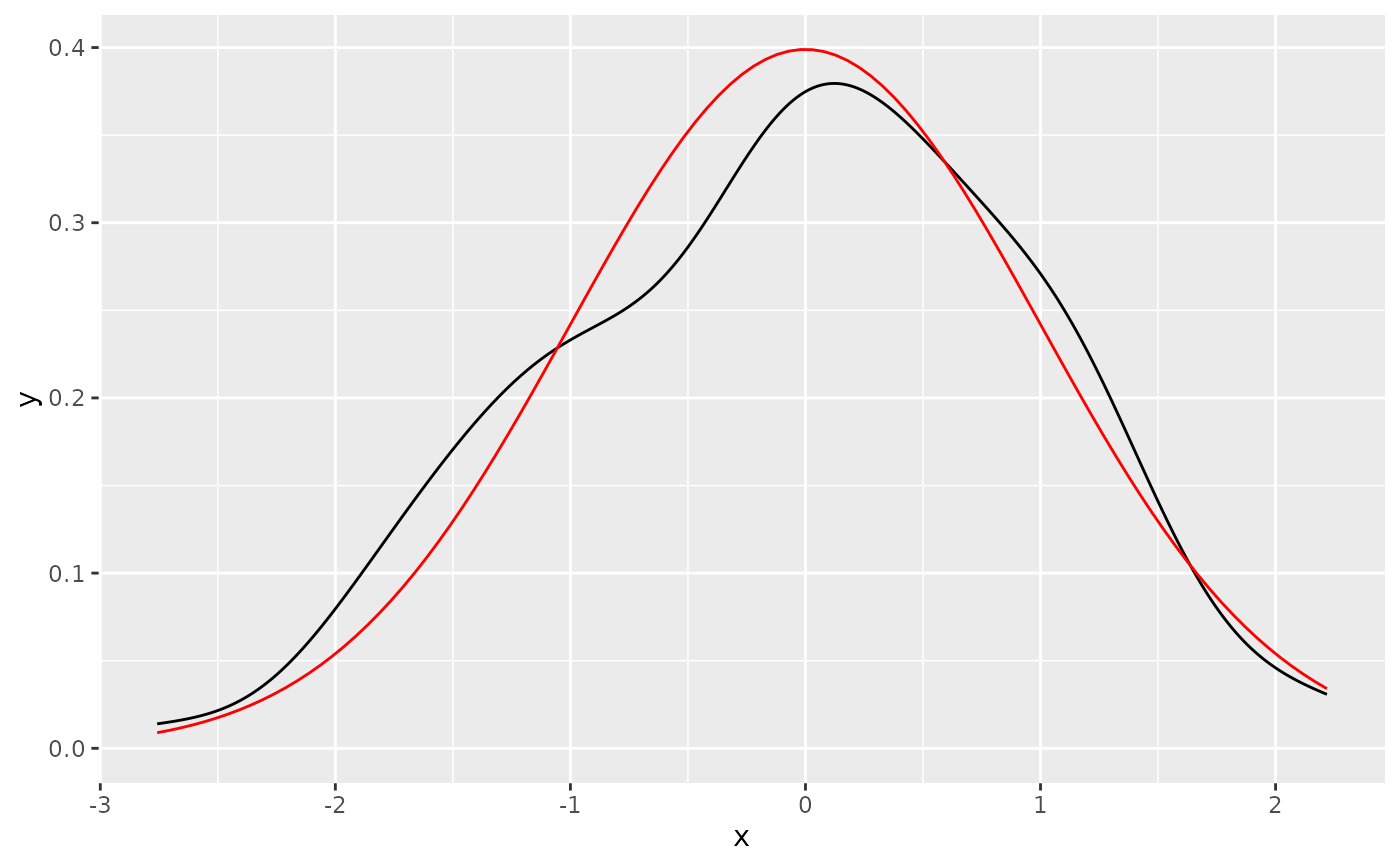# To plot functions without data, specify range of x-axis
base <-
ggplot() +
xlim(-5, 5)

base + geom_function(fun = dnorm)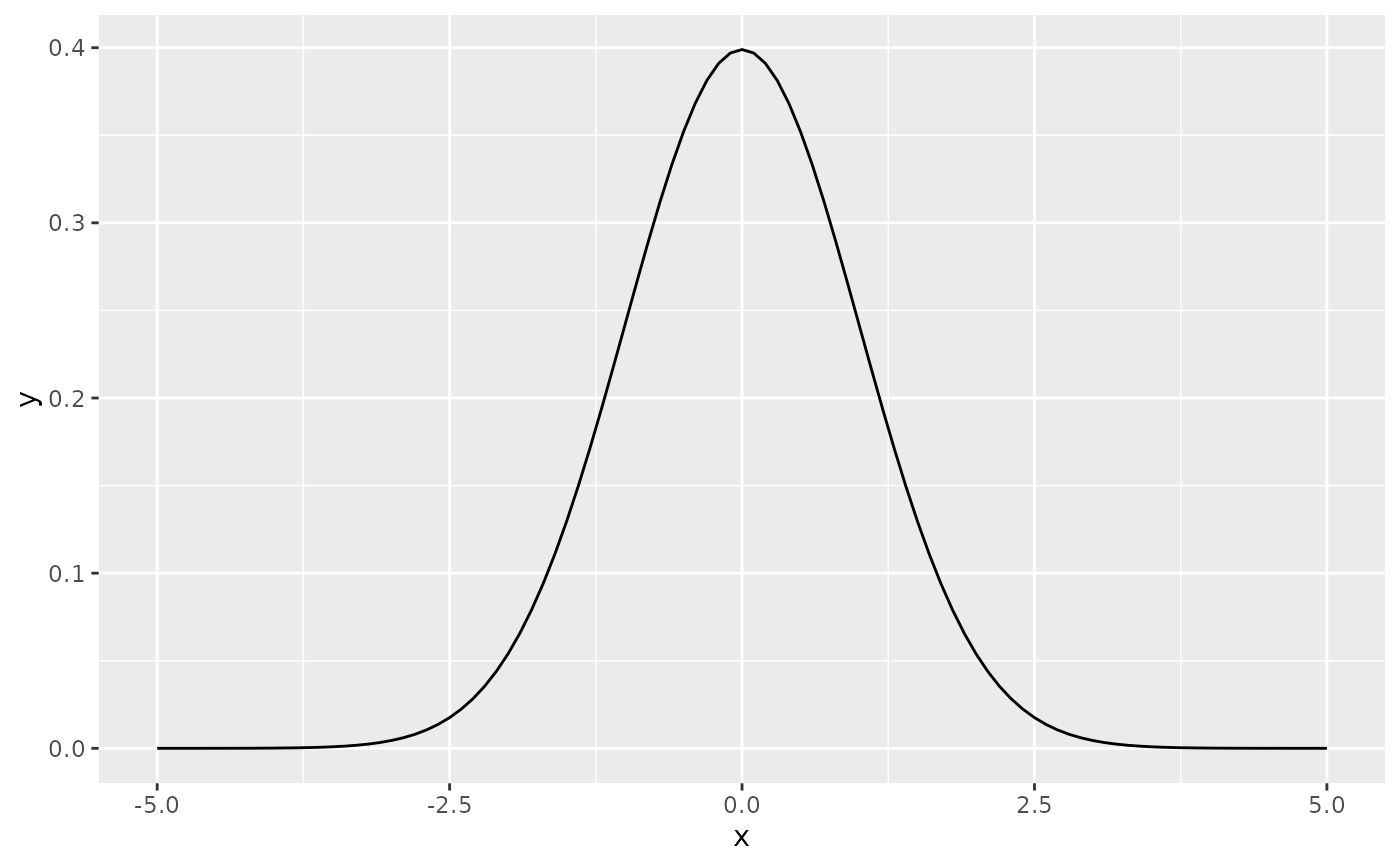base + geom_function(fun = dnorm, args = list(mean = 2, sd = .5))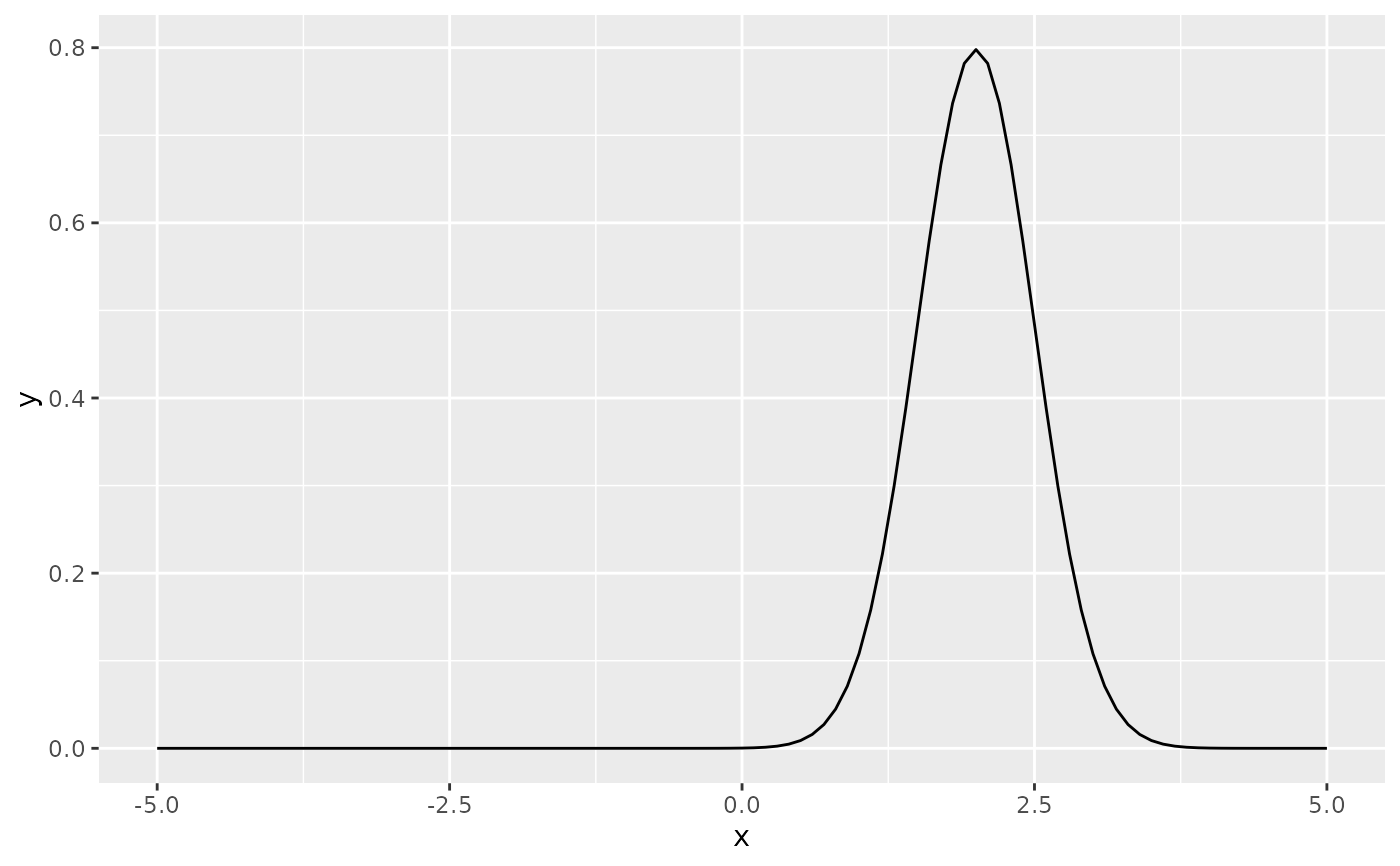# The underlying mechanics evaluate the function at discrete points
# and connect the points with lines
base + stat_function(fun = dnorm, geom = "point")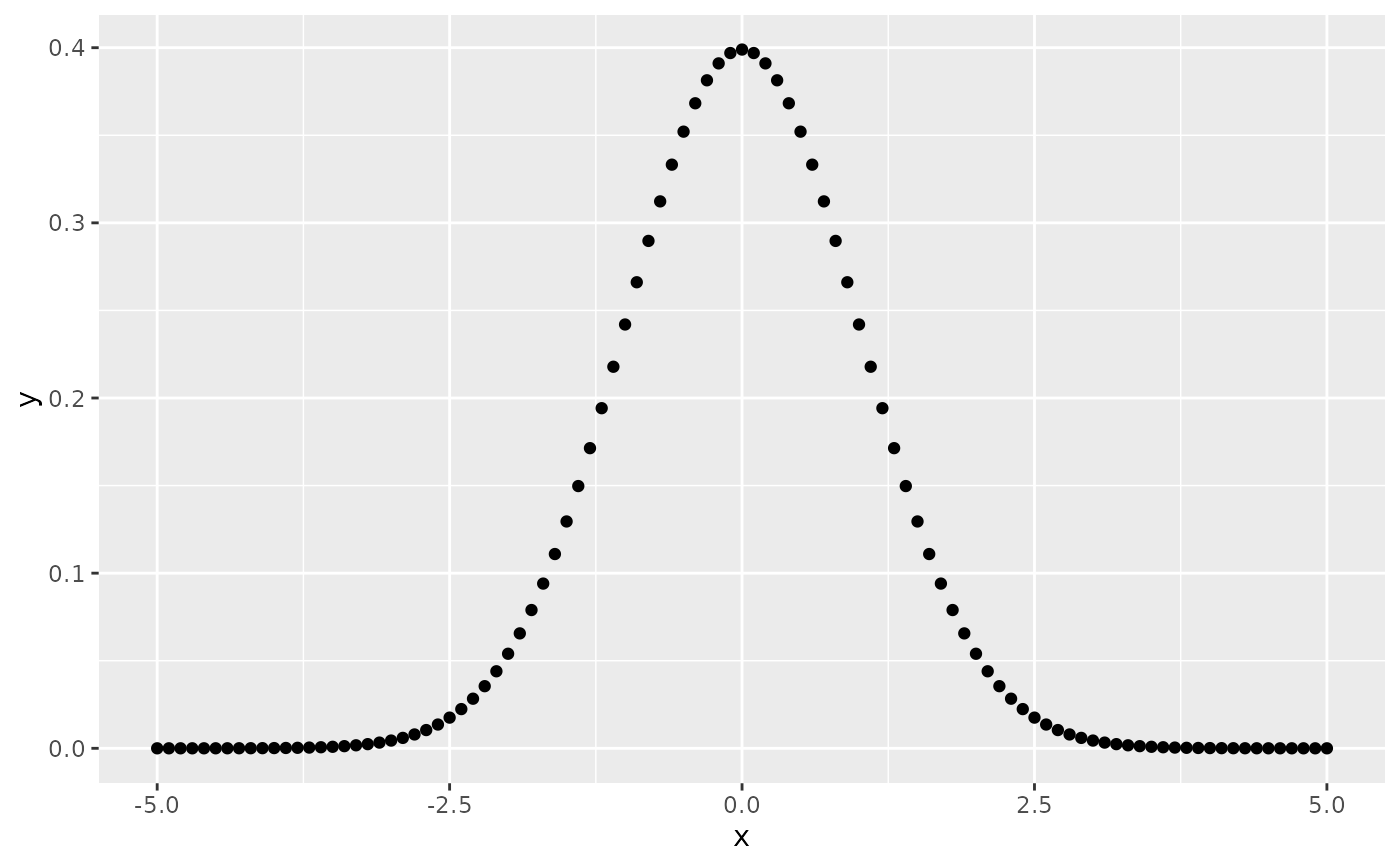base + stat_function(fun = dnorm, geom = "point", n = 20)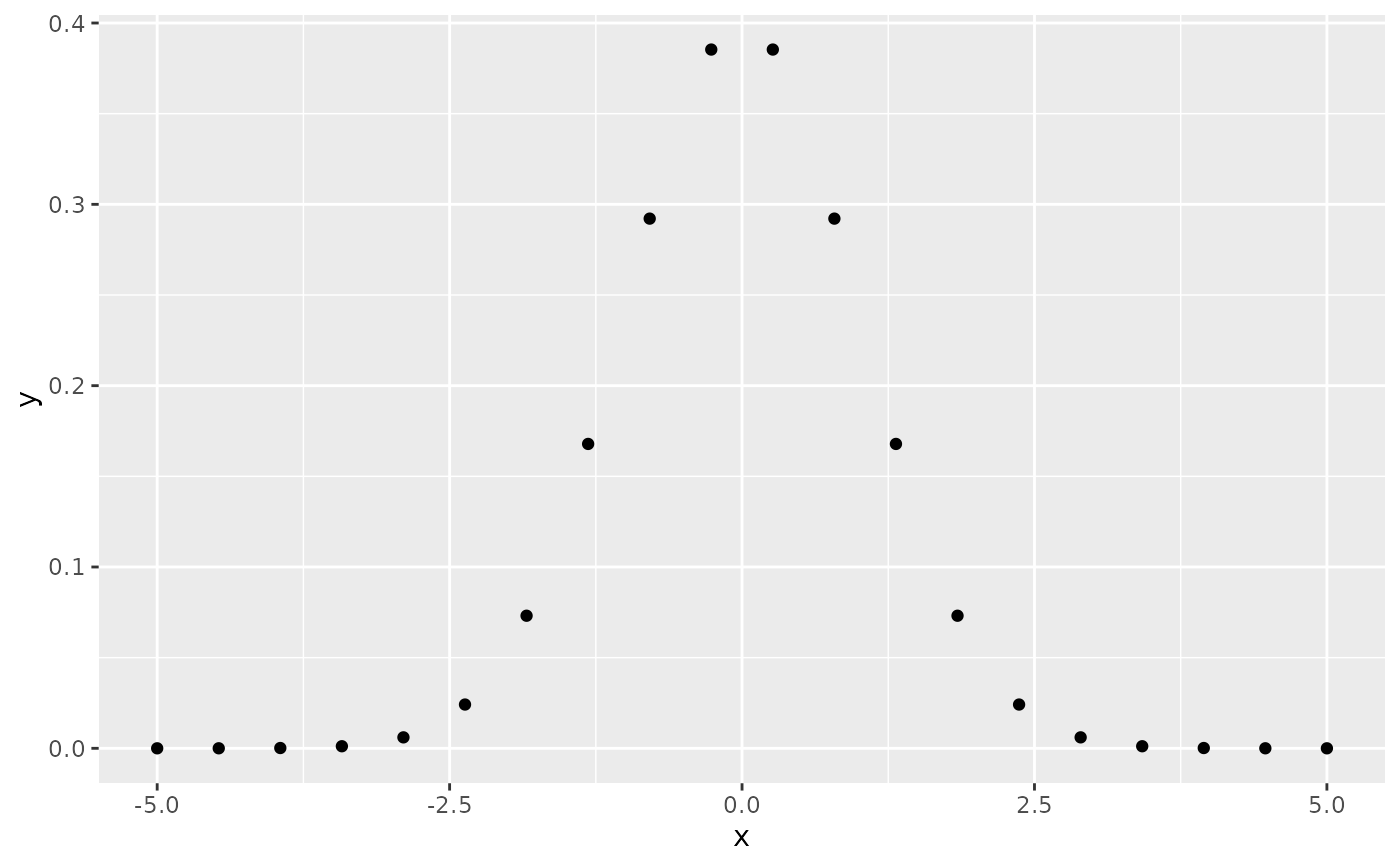base + stat_function(fun = dnorm, geom = "polygon", color = "blue", fill = "blue", alpha = 0.5)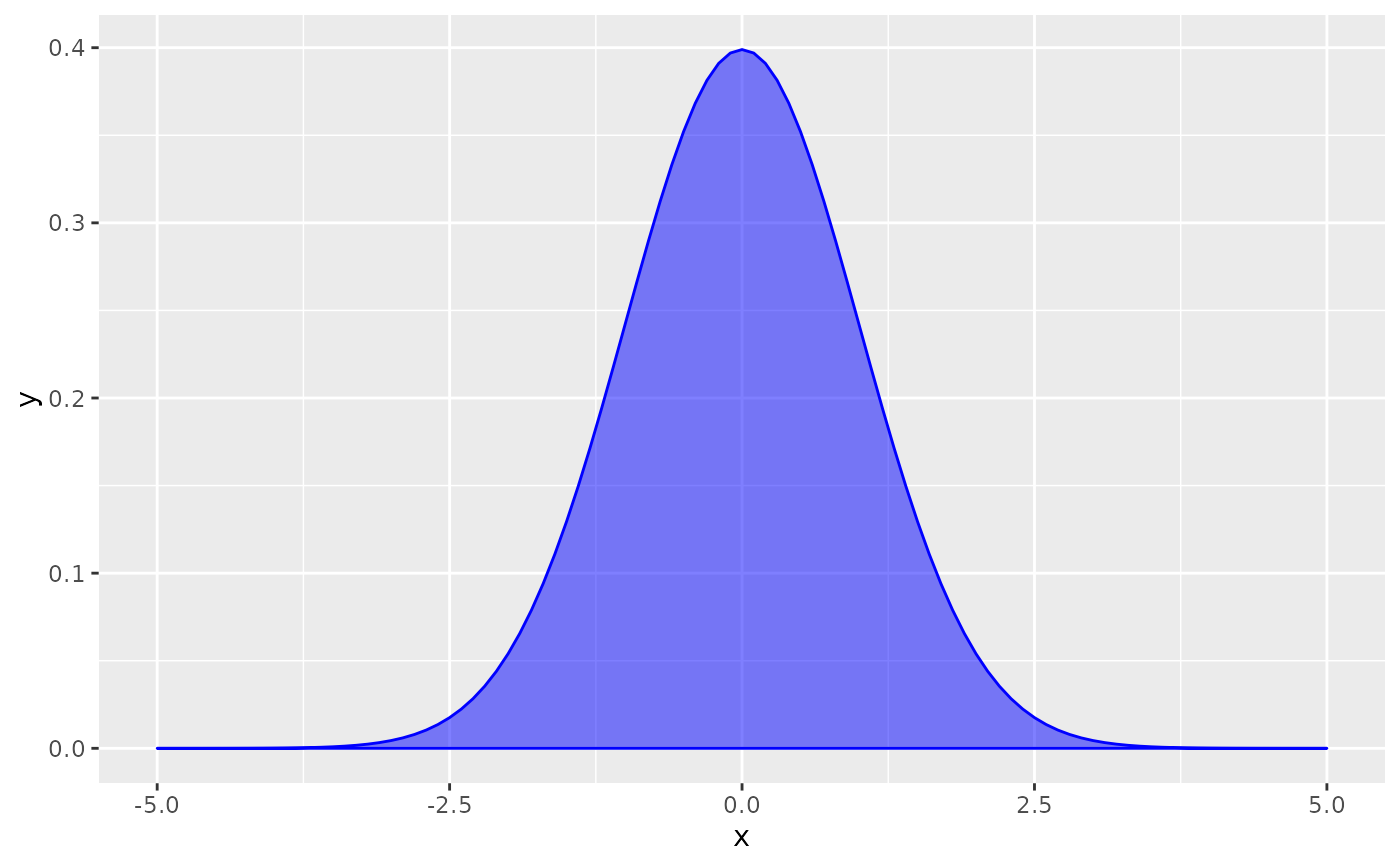base + geom_function(fun = dnorm, n = 20)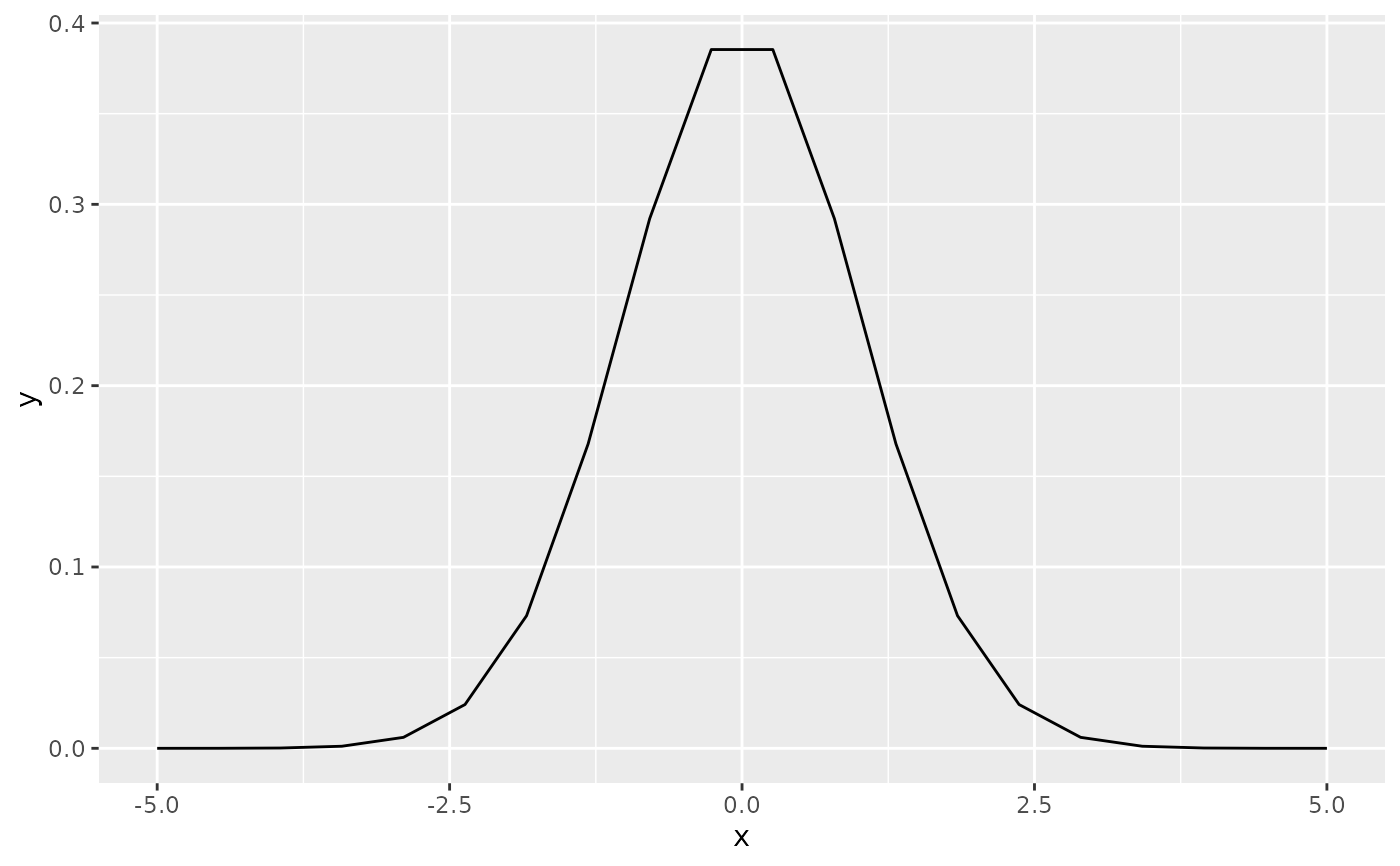# Two functions on the same plot
base +
geom_function(aes(colour = "normal"), fun = dnorm) +
geom_function(aes(colour = "t, df = 1"), fun = dt, args = list(df = 1))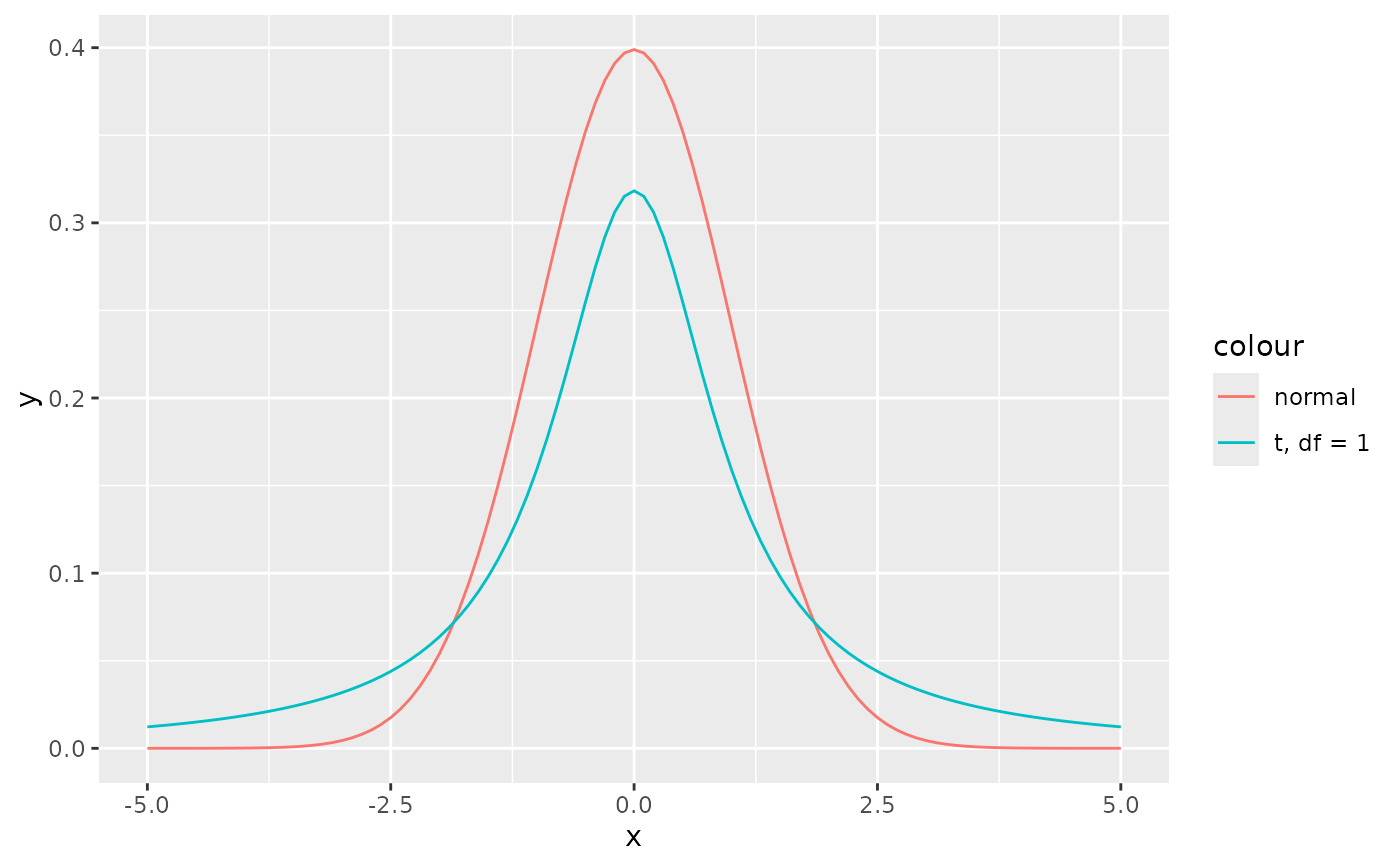# Using a custom anonymous function
base + geom_function(fun = function(x) 0.5*exp(-abs(x)))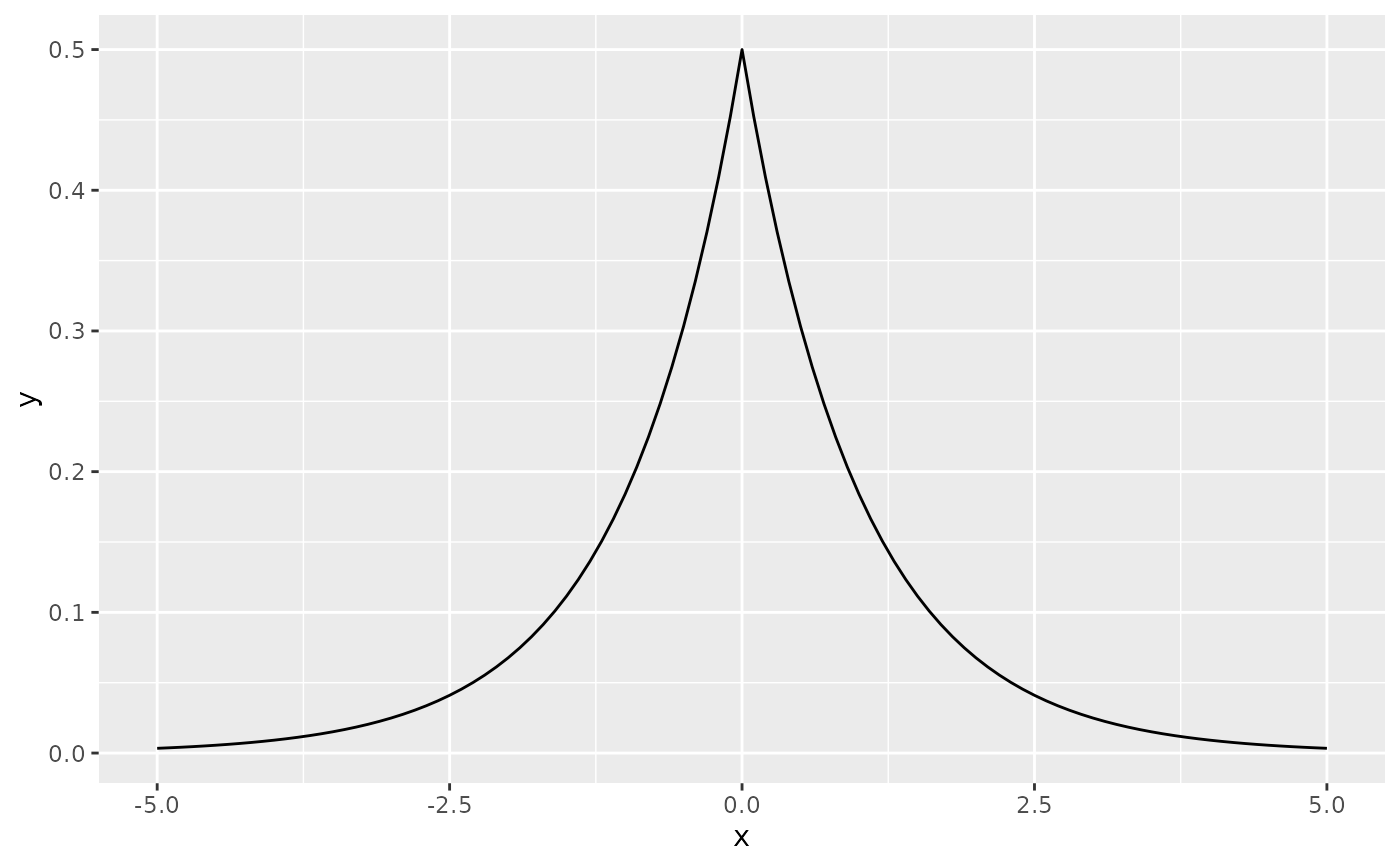base + geom_function(fun = ~ 0.5*exp(-abs(.x)))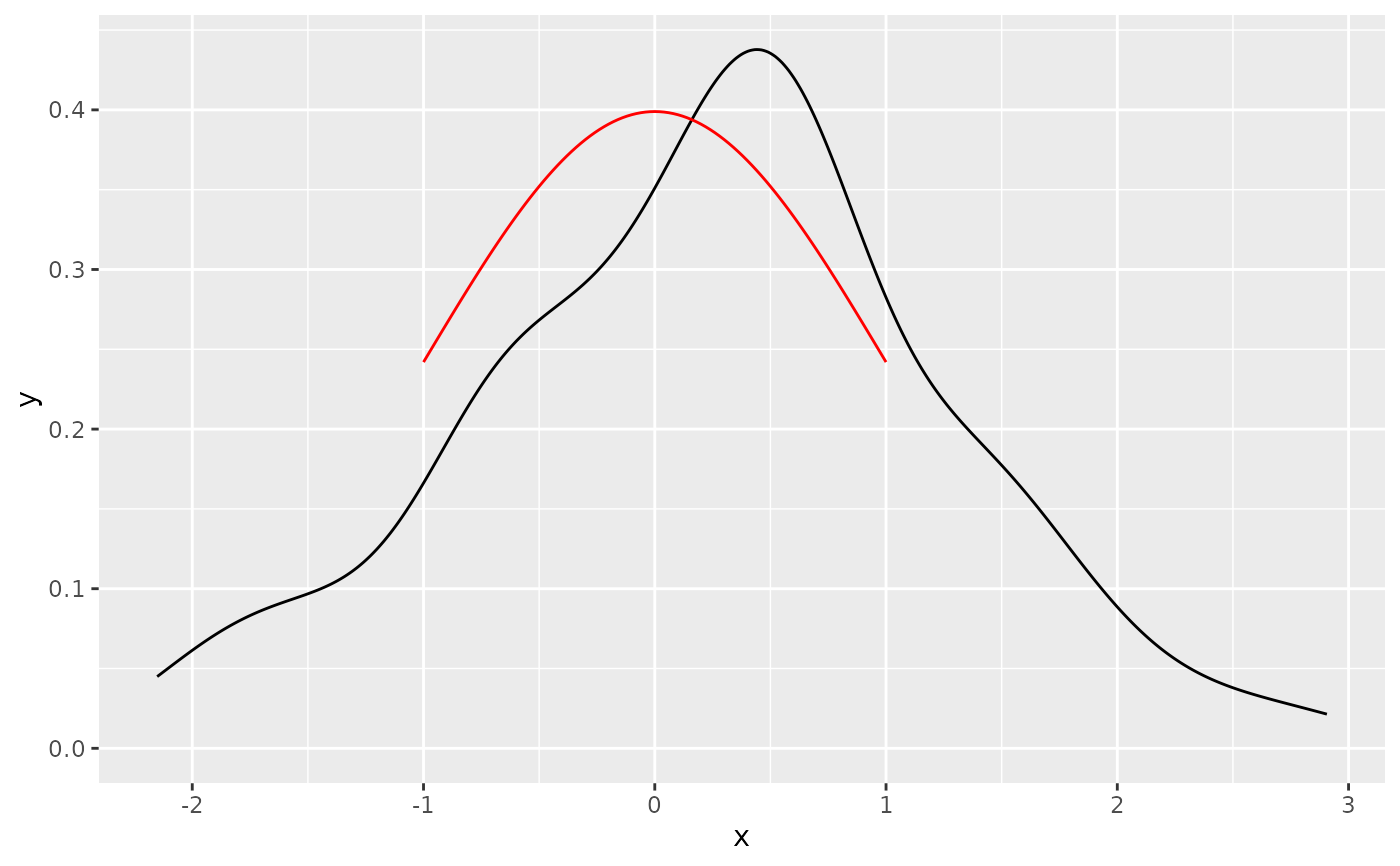# Using a custom named function
f <- function(x) 0.5*exp(-abs(x))

base + geom_function(fun = f)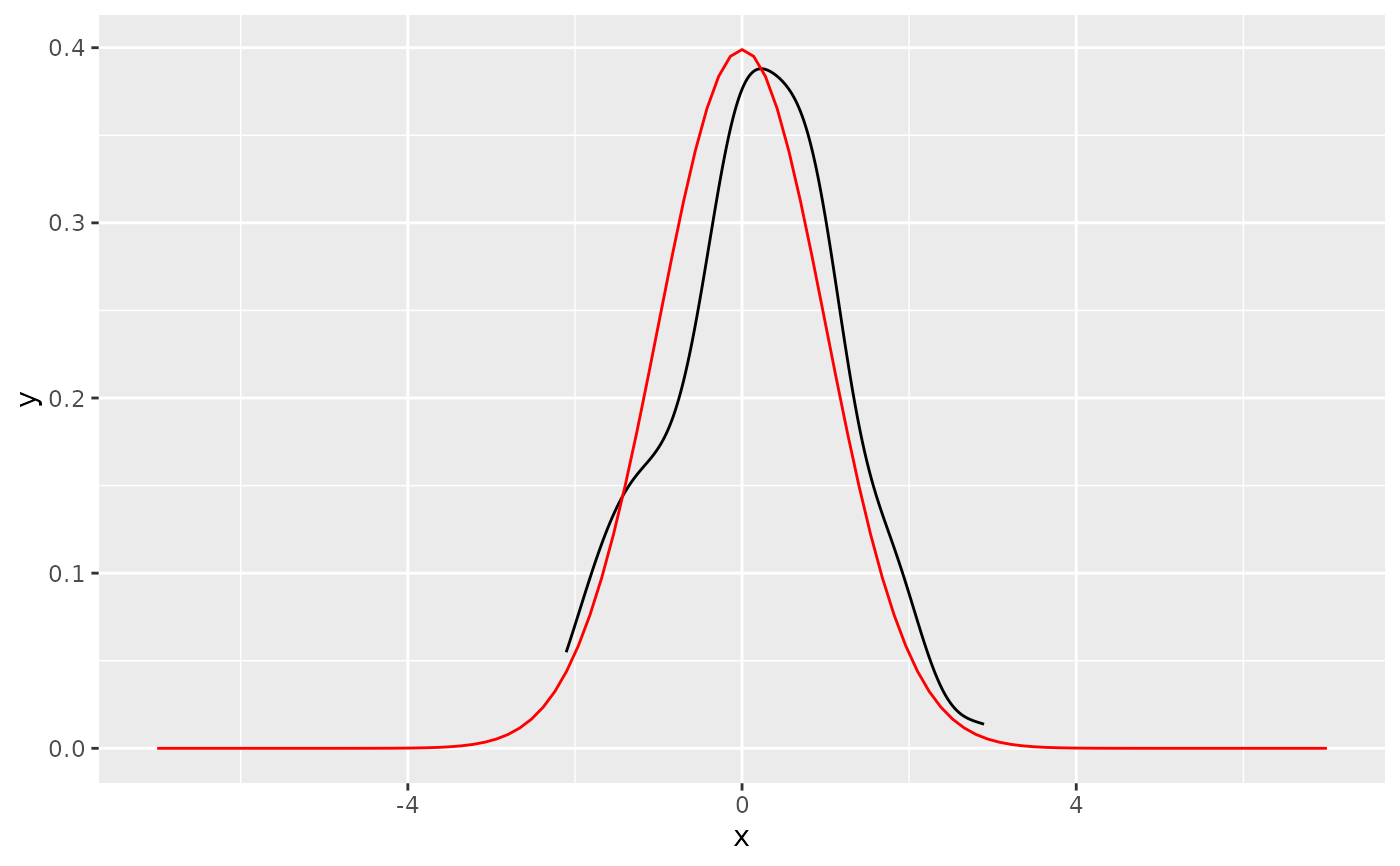# Using xlim to restrict the range of function
ggplot(data.frame(x = rnorm(100)), aes(x)) +
geom_density() +
geom_function(fun = dnorm, colour = "red", xlim=c(-1, 1))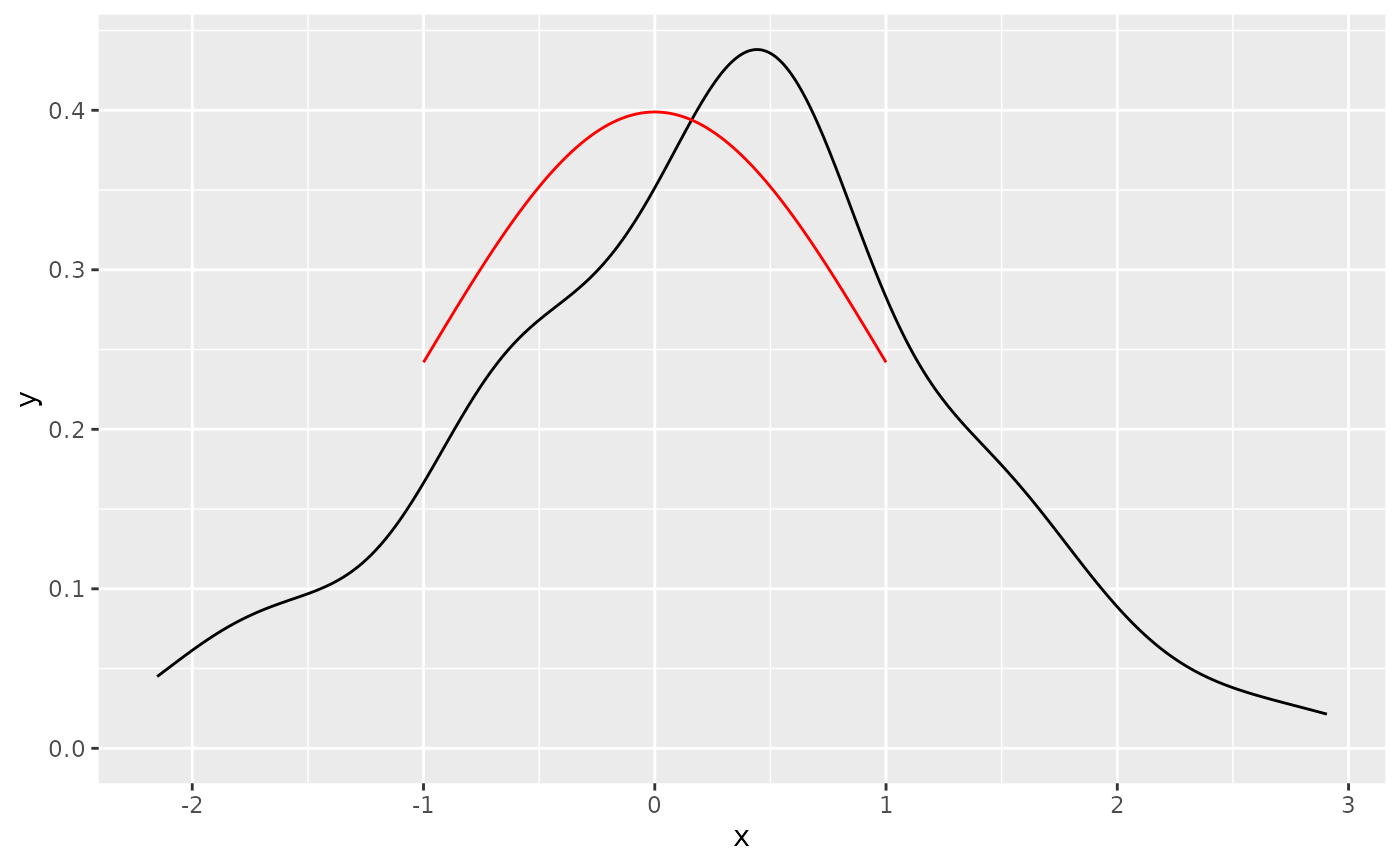# Using xlim to widen the range of function
ggplot(data.frame(x = rnorm(100)), aes(x)) +
geom_density() +
geom_function(fun = dnorm, colour = "red", xlim=c(-7, 7))